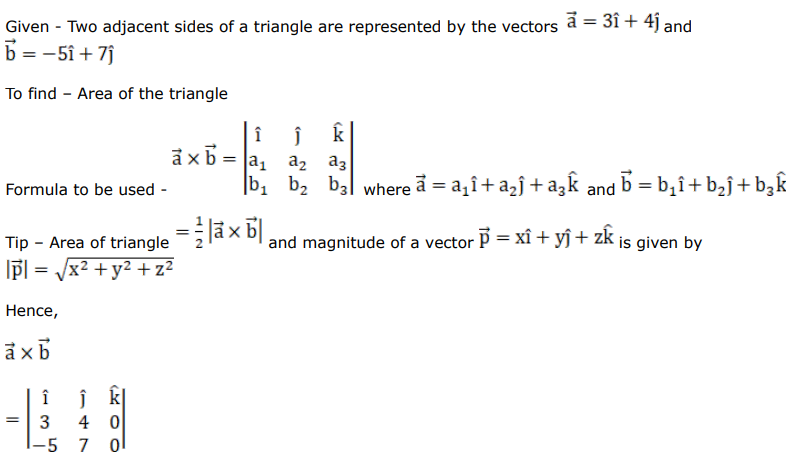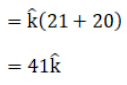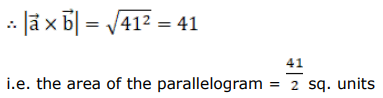# Mark against the correct answer in each of the following:

Question:

Mark $(\sqrt{)}$ against the correct answer in each of the following:

Two adjacent sides of a triangle are represented by the vectors $\overrightarrow{\mathrm{a}}=3 \hat{\mathrm{i}}+4 \hat{\mathrm{j}}$ and $\overrightarrow{\mathrm{b}}=-5 \hat{\mathrm{i}}+7 \hat{\mathrm{j}}$ The area of the triangle is

A. 41 sq units

B. 37 sq units

C. $\frac{41}{2}$ sq units

D. none of these

Solution: